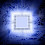## Featured post

### Top 5 books to refer for a VHDL beginner

VHDL (VHSIC-HDL, Very High-Speed Integrated Circuit Hardware Description Language) is a hardware description language used in electronic des...

## Tuesday 13 December 2011

### Finite State Machine (FSM) Coding In VHDL

There is a special Coding style for State Machines in VHDL as well as in Verilog.
Let us consider below given state machine which is a “1011” overlapping sequence detector. Output becomes ‘1’ when sequence is detected in state S4 else it remains ‘0’ for other states.
VHDL Code for FSM:
library IEEE;
use IEEE.STD_LOGIC_1164.ALL;
--Sequence detector for detecting the sequence "1011".
--Overlapping type.
entity seq_det is
port(   clk   : in std_logic;      --clock signal
reset : in std_logic;      --reset signal
S_in  : in std_logic;      --serial bit Input sequence
S_out : out std_logic);    -- Output
end seq_det;
architecture Behavioral of seq_det is
--Defines the type for states in the state machine
type state_type is (S0,S1,S2,S3,S4);
--Declare the signal with the corresponding state type.
signal Current_State, Next_State : state_type;
begin
-- Synchronous Process
process(clk)
begin
if( reset = '1' ) then                 --Synchronous Reset
Current_State <= 'S0';
elsif (clk'event and clk = '1') then   --Rising edge of Clock
Current_State <= Next_State
end if;
end process;
-- Combinational Process
Process(Current_State, S_in)
begin
case Current_State is
when S0 =>
S_out <= '0';
if ( s_in = '0' ) then
Next_State <= S0;
else
Next_State <= S1;
end if;
when S1 =>
S_out <= '1';
if ( S_in = '0' ) then
Next_State <= S3;
else
Next_State <= S2;
end if;
when S2 =>
S_out <= '0';
if ( S_in = '0' ) then
Next_State <= S0;
else
Next_State <= S3;
end if;
when S3 =>
S_out <= '1';
if (S_in = '0' ) then
Next_State <= S2;
else
Next_State <= S4;
end if;
when S4 =>
S_out <= '1';
if ( S_in = '0' ) then
Next_State <= S2;
else
Next_State <= S1;
end if;
when others =>
NULL;
end case;
end if;
end process;

1.superb :)
i totally understand the programming.
thanks a lot.

1.totally right!

2.3.helped me alot, thanks :)

4.was in search of it..thank u..:)

5.Hi, Where is the "output state" logic defined to detect the sequence "1011"

1.Thanks, there was a typo in the code... We updated it.

Regards,
Team VLSI Encyclopedia

6.very good

1.Team Vlsiencyclopedia30 October 2013 at 03:02

Thanks for appreciation :)

7.after "end case;", there is an "end if;" too much, i think

8.thank you !

9.Here the second process will not be executed if the s_in in the first clock cycle is 0. The sate machine will not move forward as processes react to only events and there will never be an event on Current_State. Sorry if i am wrong.

1.Vamsi, Thanks for writing.
You are correct, the sensitivity list should also contain input s_in.

10.why is the S_out for states S1 and S3 1? Should they not be 0?

11.If suppose we draw a mealy FSM for this detector, I guess we would be saving one extra state as instead of going from S3 to S4 the FSM can go to the state S1, for detecting the overlapping sequence 1011, the last digit 1 can serve as the beginning of new sequence 1011, am I right?

1.Yes you are right... the melay implementation will save 1 state as the output of melay is function of present state and value of inputs.

12.How can I run this code in Quartus II?

1.Please refer below guide for Quartus!!
https://goo.gl/FGO8Ow

Thanks,
Team VLSI Encyclopedia

Please provide valuable comments and suggestions for our motivation. Feel free to write down any query if you have regarding this post.